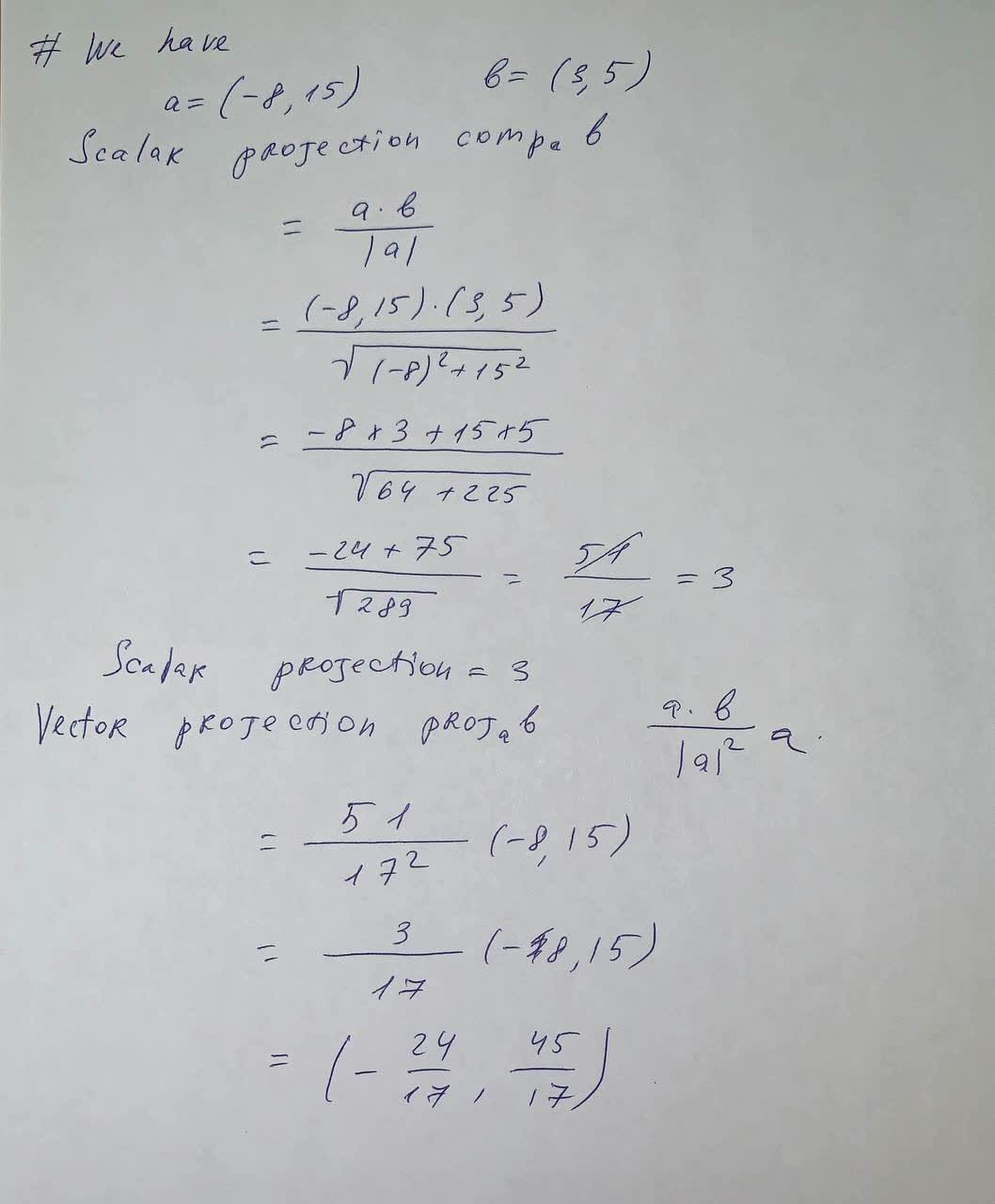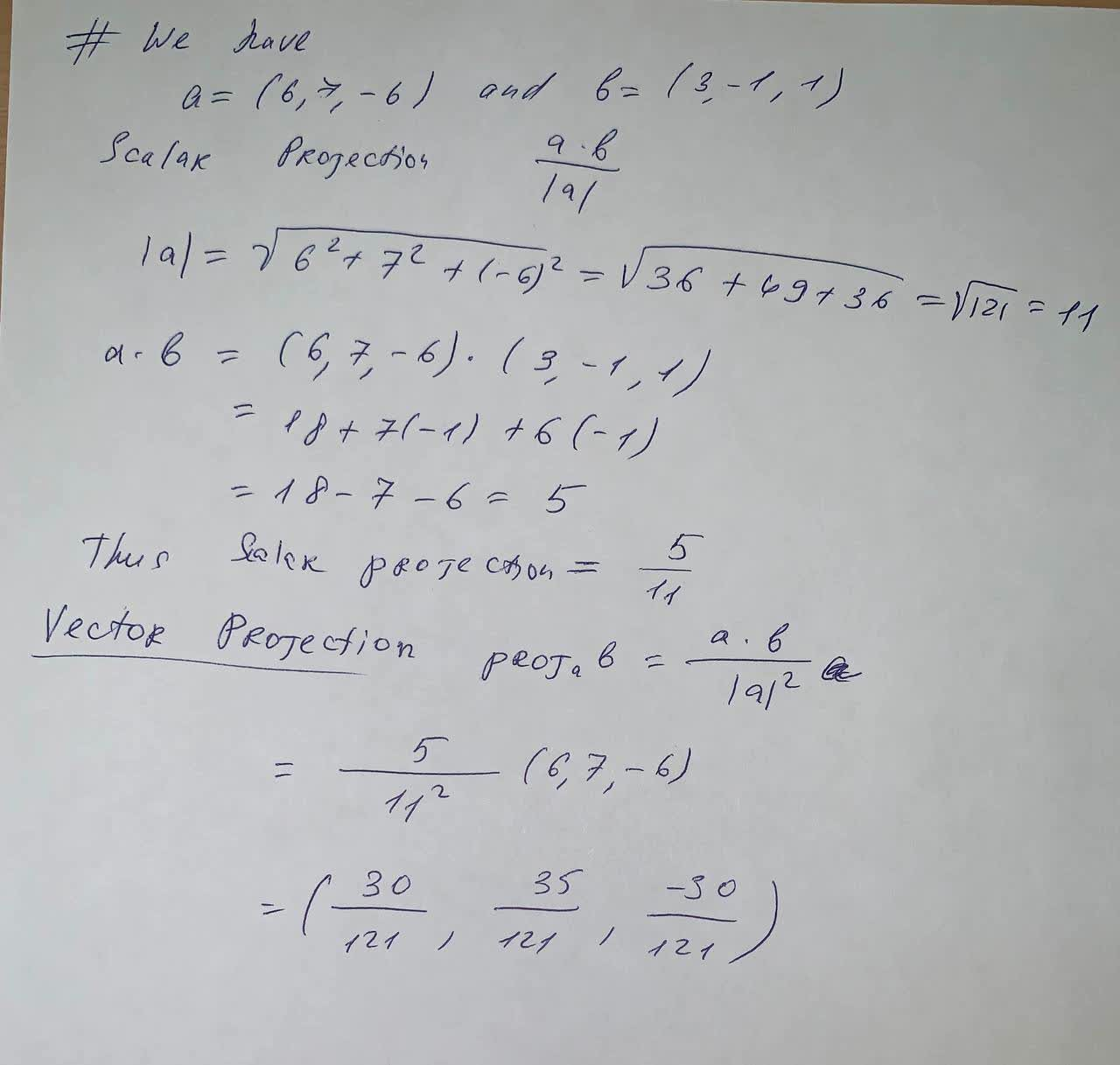Question# Find the scalar and vector projections of b onto a a=(-8,15) , b=(3,5) Find the scalar and vector projections of b onto a a=(6,7,-6) b=(3,-1,1)

Vectors
ANSWEREDFind the scalar and vector projections of b onto a
a=(-8,15) , b=(3,5)
Find the scalar and vector projections of b onto a
a=(6,7,-6) b=(3,-1,1)